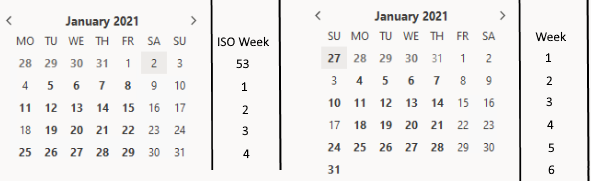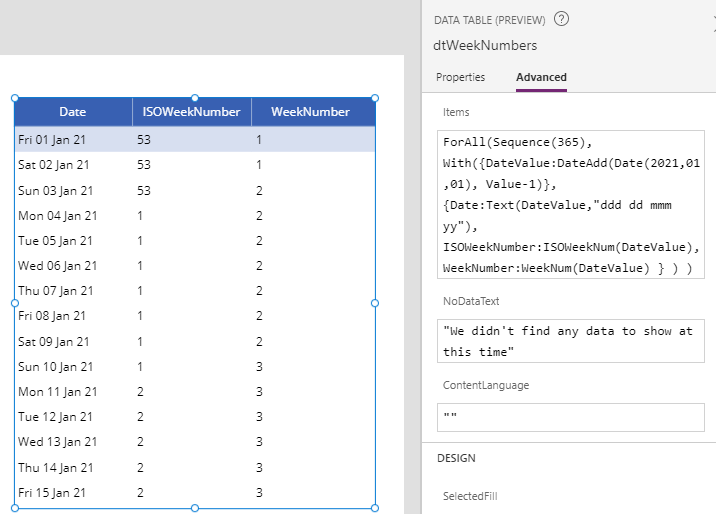Dates - How to get the week number for a given date

A common requirement is to calculate week numbers. Up till now, this task could be difficult to accomplish but now, Power Apps introduces the WeekNum and ISOWeekNum functions to help simplify this task. This post describes how these two new functions work.

Up till now, calculating week numbers relied upon custom formula. There are now 2 functions that we can call to return the week number for an input date - WeekNum and ISOWeekNum. This post describes how these functions work.

For the complete details, we can refer to the official documentation for these functions beneath:

What's the difference between Week Numbers and ISO Week Numbers?

There are different standards that define how weeks are numbered. By calling either the WeekNum or ISOWeekNum functions, we can determine a week number that matches our target scheme.

The "ISO week date" system is the common standard that many countries have adopted. ISO week 1 starts in the week where the first Thursday falls, and interestingly, the 4th January always falls into Week 1. An important characteristic of the ISO system is that the start of week begins on Monday.

There are many countries that do not follow this standard, such as the US, Canada, and Australia. These countries work on the basis that the start of a week begins on Sunday.

The image beneath illustrates the week number and ISO week numbers for the start of 2021. Notice how with standard week numbering, week 1 corresponds with the week that contains 1st January.How to the call the the ISOWeekNum and WeekNum functions

The ISOWeekNum function accepts an input date and returns the ISO week number. The WeekNum function accepts an input date and an optional argument that defines the start of week.

To demonstrate these two functions, the formula beneath generates the Week Number and ISO Week Numbers for each day in 2021.

ForAll(Sequence(365),
{Date:Text(DateValue,"ddd dd mmm yy"),
ISOWeekNumber:ISOWeekNum(DateValue),
WeekNumber:WeekNum(DateValue)
}
)
)
The screenshot beneath illustrates the output of this formula when we apply it to the Items property of a data table. We can verify here how week numbers correspond with the week numbers that are highlighted in the calendar screenshot above.The screenshot beneath illustrates the output of these functions as applied to the last week in December 2020. This highlights another characteristic of the ISO week numbering system - all 7 days in a week will always share the same week number. With the standard week numbering system, notice how for the week beginning Sun 27 December 2020, 5 days fall into week 53 and 2 days fall into week 1.How to change the start day of the week

It's possible to change the start day of the week by passing a StartOfWeek enumeration value to the WeekNum function.

As a demonstration, several middle eastern countries start the working week on a Saturday. Here's a formula that extends our previous example to return a week number that starts on Saturday.

ForAll(Sequence(365),
{Date:Text(DateValue,"ddd dd mmm yy"),
ISOWeekNum:ISOWeekNum(DateValue),
WeekNum:WeekNum(DateValue),
WeekNumSat:WeekNum(DateValue, Saturday)
}
)
)
The screenshot beneath highlights the output of this formula.Conclusion

A common requirement is to calculate week numbers. This post described how to accomplish this task with the  WeekNum and ISOWeekNum functions.